International
Tables for
Crystallography
Volume B
Reciprocal space
Edited by U. Shmueli

International Tables for Crystallography (2006). Vol. B. ch. 1.2, p. 22   | 1 | 2 |

## Section 1.2.12.1. The Gram–Charlier expansion

P. Coppensa*

aDepartment of Chemistry, Natural Sciences & Mathematics Complex, State University of New York at Buffalo, Buffalo, New York 14260-3000, USA
Correspondence e-mail: coppens@acsu.buffalo.edu

#### 1.2.12.1. The Gram–Charlier expansion

| top | pdf |

The three-dimensional Gram–Charlier expansion, introduced into thermal-motion treatment by Johnson & Levy (1974), is an expansion of a function in terms of the zero and higher derivatives of a normal distribution (Kendall & Stuart, 1958). If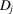is the operator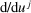,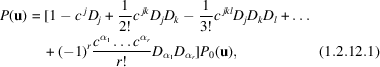where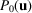is the harmonic distribution,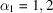or 3, and the operator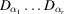is the rth partial derivative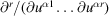. Summation is again implied over repeated indices.

The differential operators D may be eliminated by the use of three-dimensional Hermite polynomials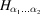defined, by analogy with the one-dimensional Hermite polynomials, by the expression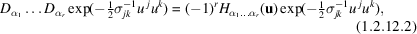which gives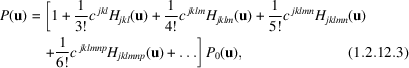where the first and second terms have been omitted since they are equivalent to a shift of the mean and a modification of the harmonic term only. The permutations of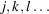here, and in the following sections, include all combinations which produce different terms.

The coefficients c, defined by (1.2.12.1)and (1.2.12.2), are known as the quasimoments of the frequency function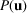(Kutznetsov et al., 1960). They are related in a simple manner to the moments of the function (Kendall & Stuart, 1958) and are invariant to permutation of indices. There are 10, 15, 21 and 28 components of c for orders 3, 4, 5 and 6, respectively. The multivariate Hermite polynomials are functions of the elements of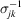and of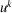, and are given in Table 1.2.12.1for orders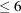(IT IV, 1974; Zucker & Schulz, 1982).

 Table 1.2.12.1| top | pdf | Some Hermite polynomials (Johnson & Levy, 1974; Zucker & Schulz, 1982)
 H(u) = 1 Hj(u) = wj Hjk(u) = wjwk − pjk Hjkl(u) = wjwkwl − (wjpkl + wkplj + wlpjk) = wjwkwl − 3w( jpkl) Hjklm(u) = wjwkwlwm − 6w( jwkplm) + 3pj( kplm) Hjklmn(u) = wjwkwlwmwn − 10w( lwmwnpjk) + 15w( npjkplm) Hjklmnp(u) = wjwkwlwmwnwp − 15w( jwkwlwmpjk) + 45w( jwkplmpnp) − 15pj( kplmpnp) where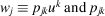are the elements of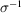, defined in expression (1.2.10.2). Indices between brackets indicate that the term is to be averaged over all permutations which produce distinct terms, keeping in mind that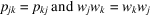as illustrated for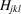.

The Fourier transform of (1.2.12.3)is given by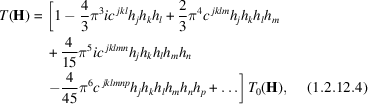where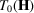is the harmonic temperature factor.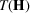is a power-series expansion about the harmonic temperature factor, with even and odd terms, respectively, real and imaginary.

### ReferencesInternational Tables for X-ray Crystallography (1974). Vol. IV. Birmingham: Kynoch Press. (Present distributor Kluwer Academic Publishers, Dordrecht.)Google ScholarJohnson, C. K. & Levy, H. A. (1974). Thermal motion analysis using Bragg diffraction data. In International tables for X-ray crystallography (1974), Vol. IV, pp. 311–336. Birmingham: Kynoch Press. (Present distributor Kluwer Academic Publishers, Dordrecht.)Google ScholarKendall, M. G. & Stuart, A. (1958). The advanced theory of statistics. London: Griffin.Google ScholarKutznetsov, P. I., Stratonovich, R. L. & Tikhonov, V. I. (1960). Theory Probab. Its Appl. (USSR), 5, 80–97.Google ScholarZucker, U. H. & Schulz, H. (1982). Statistical approaches for the treatment of anharmonic motion in crystals. I. A comparison of the most frequently used formalisms of anharmonic thermal vibrations. Acta Cryst. A38, 563–568.Google Scholar